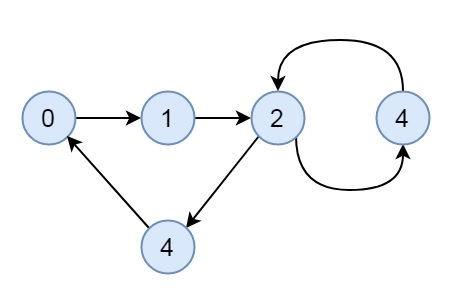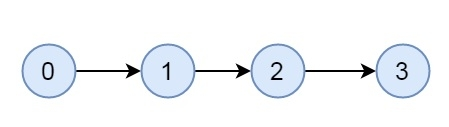# Check if a given directed graph is strongly connected in C++

Suppose we have a graph. We have to check whether the graph is strongly connected or not. A graph is said to be strongly connected, if any two vertices have a path between them, then the graph is connected. An undirected graph is strongly connected graph. Some undirected graph may be connected but not strongly connected. This is an example of a strongly connected graph.This is an example of a connected, but not strongly connected graph.Here we will see, how to check a graph is strongly connected or not using the following steps.

Steps

• Mark all nodes as not visited

• Start DFS traversal from any arbitrary vertex u. If the DFS fails to visit all nodes, then return false.

• Reverse all edges of the graph

• Set all vertices as not visited nodes again

• Start DFS traversal from that vertex u. If the DFS fails to visit all nodes, then return false. otherwise true.

## Example

Live Demo

#include <iostream>
#include <list>
#include <stack>
using namespace std;
class Graph {
int V;
void dfs(int v, bool visited[]);
public:
Graph(int V) {
this->V = V;
}
~Graph() {
}
bool isStronglyConnected();
Graph reverseArc();
};
void Graph::dfs(int v, bool visited[]) {
visited[v] = true;
list<int>::iterator i;
if (!visited[*i])
dfs(*i, visited);
}
Graph Graph::reverseArc() {
Graph graph(V);
for (int v = 0; v < V; v++) {
list<int>::iterator i;
}
return graph;
}
void Graph::addEdge(int u, int v) {
}
bool Graph::isStronglyConnected() {
bool visited[V];
for (int i = 0; i < V; i++)
visited[i] = false;
dfs(0, visited);
for (int i = 0; i < V; i++)
if (visited[i] == false)
return false;
Graph graph = reverseArc();
for(int i = 0; i < V; i++)
visited[i] = false;
graph.dfs(0, visited);
for (int i = 0; i < V; i++)
if (visited[i] == false)
return false;
return true;
}
int main() {
Graph graph(5);
graph.isStronglyConnected()? cout << "This is strongly connected" : cout << "This is not strongly connected";
}

## Output

This is strongly connected

Updated on: 22-Oct-2019

188 Views